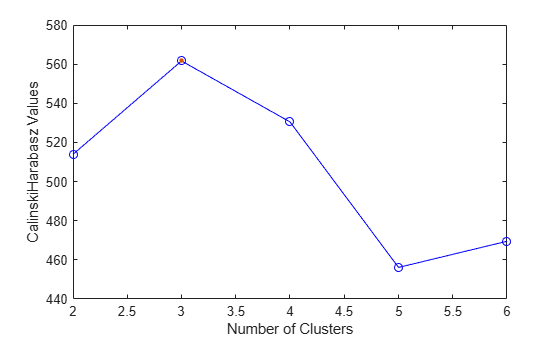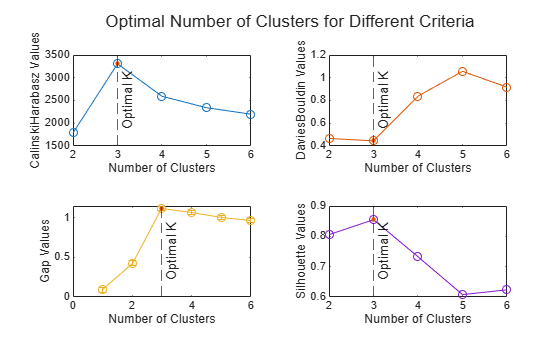# plot

Plot clustering evaluation object criterion values

## Syntax

``plot(evaluation)``
``h = plot(evaluation)``

## Description

example

````plot(evaluation)` displays a plot of the criterion values versus the number of clusters, based on the values in the clustering evaluation object `evaluation`.```

example

````h = plot(evaluation)` returns a `Line` object. Use this object to inspect and adjust the properties of the plot line. For a list of properties, see Line Properties.```

## Examples

collapse all

Plot the criterion values versus the number of clusters for each clustering solution stored in a clustering evaluation object.

Load the `fisheriris` data set. The data contains length and width measurements from the sepals and petals of three species of iris flowers.

`load fisheriris`

Create a clustering evaluation object. Cluster the data using `kmeans`, and evaluate the optimal number of clusters using the Calinski-Harabasz criterion.

```rng("default") % For reproducibility evaluation = evalclusters(meas,"kmeans","CalinskiHarabasz","KList",1:6);```

Plot the Calinski-Harabasz criterion values for each number of clusters tested.

`plot(evaluation)`The plot shows that the highest Calinski-Harabasz value occurs at three clusters, suggesting that the optimal number of clusters is three.

Cluster data using each of the four clustering evaluation criteria. For each criterion, create a plot of the criterion values and indicate the optimal number of clusters.

Generate sample data containing random numbers from three multivariate distributions with different parameter values.

```rng("default") % For reproducibility n = 200; mu1 = [2 2]; sigma1 = [0.9 -0.0255; -0.0255 0.9]; mu2 = [5 5]; sigma2 = [0.5 0; 0 0.3]; mu3 = [-2 -2]; sigma3 = [1 0; 0 0.9]; X = [mvnrnd(mu1,sigma1,n); ... mvnrnd(mu2,sigma2,n); ... mvnrnd(mu3,sigma3,n)];```

Cluster the data using `kmeans`, and evaluate the optimal number of clusters using the Calinski-Harabasz, Davies-Bouldin, gap, and silhouette criteria.

```calinskiEvaluation = evalclusters(X,"kmeans","CalinskiHarabasz", ... "KList",1:6); daviesEvaluation = evalclusters(X,"kmeans","DaviesBouldin", ... "KList",1:6); gapEvaluation = evalclusters(X,"kmeans","gap","KList",1:6); silhouetteEvaluation = evalclusters(X,"kmeans","silhouette", ... "KList",1:6);```

For each clustering evaluation object, plot the criterion values for the number of proposed clusters. In each plot, change the color of the plot line and add a vertical line indicating the optimal number of clusters.

```t = tiledlayout(2,2); title(t,"Optimal Number of Clusters for Different Criteria") colors = lines(4); % Calinski-Harabasz Criterion Plot nexttile h1 = plot(calinskiEvaluation); h1.Color = colors(1,:); hold on xline(calinskiEvaluation.OptimalK,"--","Optimal K", ... "LabelVerticalAlignment","middle") hold off % Davies-Bouldin Criterion Plot nexttile h2 = plot(daviesEvaluation); h2.Color = colors(2,:); hold on xline(daviesEvaluation.OptimalK,"--","Optimal K", ... "LabelVerticalAlignment","middle") hold off % Gap Criterion Plot nexttile h3 = plot(gapEvaluation); h3.Color = colors(3,:); hold on xline(gapEvaluation.OptimalK,"--","Optimal K", ... "LabelVerticalAlignment","middle") hold off % Silhouette Criterion Plot nexttile h4 = plot(silhouetteEvaluation); h4.Color = colors(4,:); hold on xline(silhouetteEvaluation.OptimalK,"--","Optimal K", ... "LabelVerticalAlignment","middle") hold off```The four plots indicate that the optimal number of clusters is three, regardless of the clustering criterion.

## Input Arguments

collapse all

Clustering evaluation data, specified as a `CalinskiHarabaszEvaluation`, `DaviesBouldinEvaluation`, `GapEvaluation`, or `SilhouetteEvaluation` clustering evaluation object. Create a clustering evaluation object by using `evalclusters`.

## Version History

Introduced in R2013b